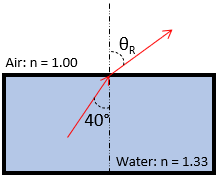## Angle of Refraction

A child underwater in a pool shines a flashlight towards the surface at a 40° angle from the vertical. What angle does the beam of light leave the pool? Note the index of refraction for water and air are 1.33 and 1.00 respectively.

HintHint 2
Snell’s Law:
$$n_isin\theta_i=n_rsin\theta_r$$$where $$n_i$$ is the incident index, $$n_r$$ is the refracted index, $$\theta_i$$ is the incident angle, and $$\theta_r$$ is the refracted angle. Snell’s Law: $$n_isin\theta_i=n_rsin\theta_r$$$
where $$n_i$$ is the incident index, $$n_r$$ is the refracted index, $$\theta_i$$ is the incident angle, and $$\theta_r$$ is the refracted angle.Thus, the refracted angle is:
$$(1.33)sin40^{\circ}=(1.00)sin\theta_r$$$$$sin\theta_r=\frac{0.855}{1.00}=0.855$$$
$$\theta_r=sin^{-1}(0.855)=58.7^{\circ}$$\$
58.7°